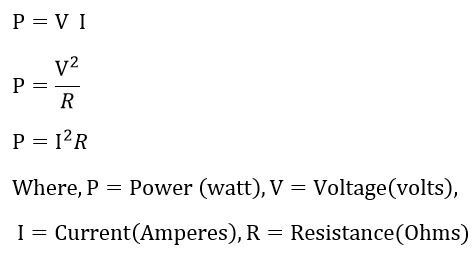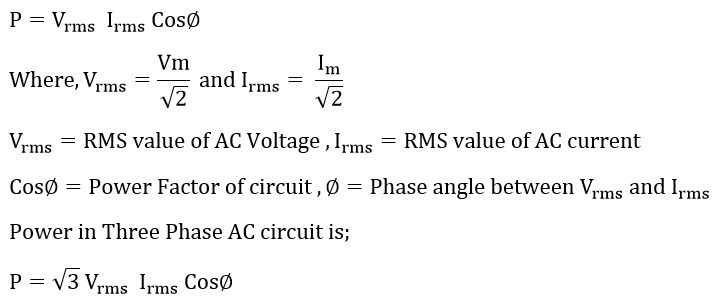Search

# What is Electric Power? Definition, Units & Types

Updated: Aug 20

### Definition:What is Power?

The rate of work done is called an electric power. Mathematically, power can be expressed as;

P = W/t

P = Power in Watts

W = Work done in Joules

t = Time in Seconds

The standard metric unit of power is watt. 1 Watt is equivalent to 1 Joule/second.

The sources of electric power are generators and batteries. The generated power is transferred through a long transmission line for end use of the power. The energy can be neither created nor it can be destroyed, however the energy can be transformed from one form to another. The electrical power can be easily converted into other power sources like heat,motion. The resistance change the electric power into heat. The motor converts electrical power into mechanical energy(motion).

The power is measured using the watt meter.

### Units of Electric Power

SI unit of power is Watt. The other units of power is microwatts (µW), miliwatt (mW), kilowatt (kW), megawatt (MW), and Gigawatt (GW). The bigger units of power is kilowatt, megawatt and gigawatt.

### Electric Power Equations

When voltage is applied to an electric circuit, current flows through the circuit. The magnitude of electric current flowing in the circuit depends on the applied voltage and resistance of the circuit. The formula of power consumed by the circuit is as given below.50 Volts applied to circuit which has 5 ohms resistance. What is the power consumed by the circuit.

I = V/R = 50/ 5 = 10 Amps.

P = VI

P= 50 x 10 = 500 Watts

The electrical energy consumed can be calculated by taking the integration of power consumed over a period of time. Energy meter records the energy consumed.

## Types of an Electric Power

There are two types of voltage source used in an electric circuit viz. AC source and Dc source and electric power can be classified into two types.

## 1. AC Power

Active or Real Power (P)

The phase relationship between voltage and current is very important for calculation of AC power. If the current flowing in the circuit has no phase difference with applied voltage, the circuit is resistive and the power consumed by the circuit is active power. The apparent power and the reactive power is zero. The active power in a single phase AC circuit can be expressed by following formula.The ratio of real power to apparent power is called power factor, and its value is between 0 and 1.The power factor of the resistive circuit is unity and it consumes only active power. The purly inductive and capacitive circuit do not consume any active power and consume only reactive power.

Reactive Power (Q)

The power consumed by the reactance of the circuit is called reactive power(Q).The reactive power is measured in volt-ampere.Apparent Power (S)

Apparent power is wattless power circuit consumes from the source. The apparent power is expressed in volt-ampere. Though apparent power is wattless power, the increased current due to reactive loads increase the power loss in transmission line and cables.### 2. DC Power

DC voltage is constant and it does not change its polarity. The DC power can be calculated by multiplying voltage and current.